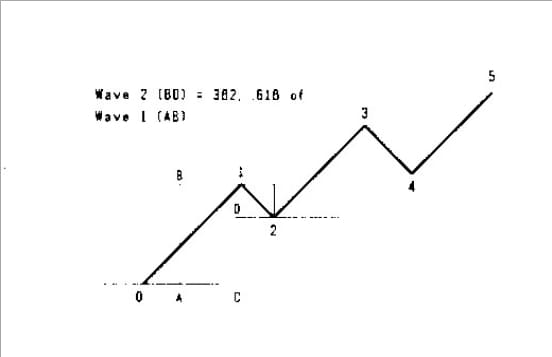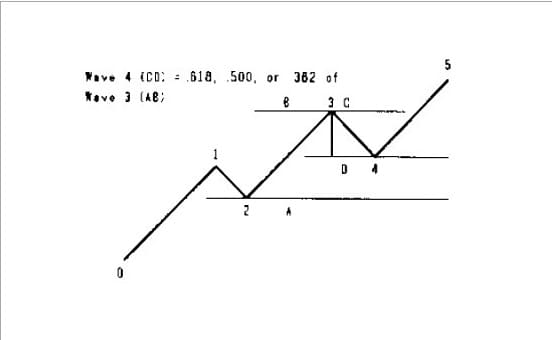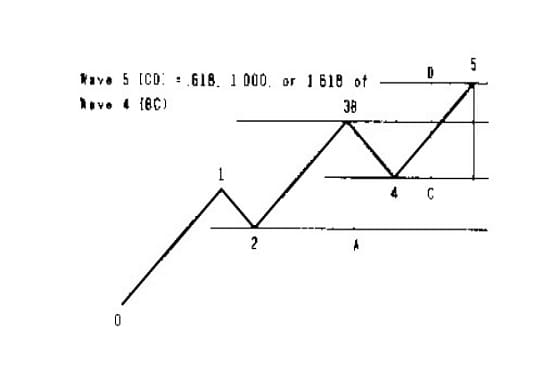# How to Use the Ratio Compass Divider By Larry Jacobs

5/5 - (1 vote)

The Elliott Wave method of market analysis is an excellent technical analysis method. The basic idea of the Elliott Wave Theory, is that a bull market has ﬁve waves up to a top followed by a down turn. Waves 1,3, and 5 are thrust waves and waves 2 and 4 are corrective waves. Two of the thrust waves are the same length. Within each of these wave patterns are smaller wave patterns. The Elliott Wave Theory states that if wave 2 is a simple correction (prices falling without interruption in the down trend), then wave 4 will be a complex correction with a 3 wave A B C movement down to wave 4.

Within this pattern, expanding waves usually will increase by the ratios .618, 1.000, 1.618. 2.618, 3.618 or 4.618. Corrections usually correct .382, .500 or .618 of the prior thrust wave. To get started you must ﬁrst set the compass to the golden ratio. To do this close the compass. Set the cross hair to the golden ratio setting on the right-hand scale. CD = .618 AB. Use this setting to project the contracting or expanding waves objectives.

### Wave 2PROCEDURE:. Place the compass point A at 0 and point B at the top of wave 1. Flip the compass. Place point C on 0 base line and mark point D. Point D to the top of wave 1 is the .382 reaction point of wave 1.

THEORY: The corrective wave 2 will usually react .382 of thrust wave one. The next common is .6 18.

### Wave 3PROCEDURE: Place compass point A on the 0 base line. Place point B at the top of wave 1. Flip the compass. Place point C at the bottom of wave 2 and mark point D. This is the projected top of wave 3.

THEORY: Thrust wave 3 will usually advance .618 of wave I beginning at the bottom of wave 2. The next common advance is 1.000 of wave 1 and the least common is 1.618 of wave 1.

### Wave 4PROCEDURE: Place the compass point A at the bottom of wave 2 and point B at the top of wave 3. Flip the compass. Place the compass point C at the top of wave 3 and mark point D. This is the .618 reaction point for wave 4.

THEORY: The corrective wave 4 is usually .618 of wave 3. the next common retracement is .500 of wave 3 and the least common retracement is .382 of wave 3.

### Wave 5PROCEDURE: Place compass point A at the bottom of wave 2. Place compass point B at the top of wave 3. Flip the compass. Place compass point C at the bottom of wave 4 and mark point D. This is the projected top of wave 5.

THEORY: The thrust wave will usually advance .618 of wave 4. The next common advancement is 1.000 of wave 4 and least common is 1.6 18 of wave 4.

There are many other ratios that can be used to project wave lengths. Some are related to the golden ratios and others are linked to the 1/2 and 1/3 points of the Gann Square. The techniques to be used with these ratios are very interesting and can be highly proﬁtable. In following issues we will go into some of the other ratios and techniques that can be used with them.